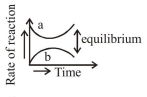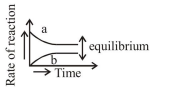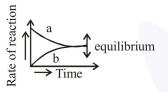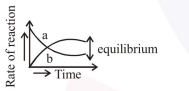# For the equilibrium A ⇌ B,

Question:

For the equilibrium $\mathrm{A} \rightleftharpoons \mathrm{B}$, the variation of the rate of the forward (a) and reverse (b) reaction with time is given by

1.2.3.4.Correct Option: , 3

Solution:

at equilibrium

$r_{a}=r_{b}$